# PHYSICS HELP!!!! PLZ!

A proton travels with a speed of 4.90 106 m/s at an angle of 64° with the direction of a magnetic field of magnitude 0.250 T in the positive x-direction.

(a) What is the magnitude of the magnetic force on the proton?

(b) What is the proton's acceleration?

givenB = 0.250Tq = 1.6 * 10-19Cv = 4.90106m/s? = 640magnitude of magnetic forceF = qvB sin?= 1.76 * 10-19Nproton acclerationradius of proton pathr = mv/qB= 1.67 * 10-27kg * 4.90106m/s/1.6 * 10^-19 * 0.250T = 20.46 * 10-8maccleration = a = v2/r= 1.17 * 108 m/s2

#### Earn Coin

Coins can be redeemed for fabulous gifts.

Similar Homework Help Questions
• ### Will rate for answer with work! A proton travels with a speed of 5.00 X 106...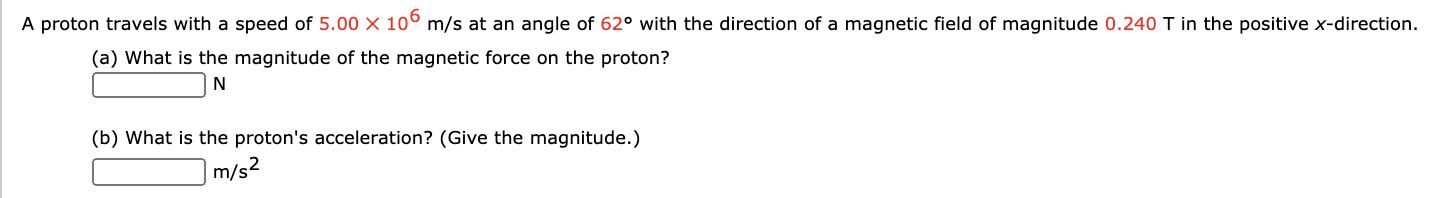Will rate for answer with work! A proton travels with a speed of 5.00 X 106 m/s at an angle of 62° with the direction of a magnetic field of magnitude 0.240 T in the positive x-direction. (a) What is the magnitude of the magnetic force on the proton? N (b) What is the proton's acceleration? (Give the magnitude.) m/s2

• ### will rate, thanks! A proton travels with a speed of 5.05 106 m/s at an angle...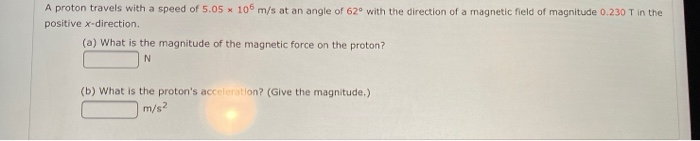will rate, thanks! A proton travels with a speed of 5.05 106 m/s at an angle of 62° with the direction of a magnetic field of magnitude 0.230 T in the positive x-direction. (a) What is the magnitude of the magnetic force on the proton? N (b) What is the proton's acceleration? (Give the magnitude.) m/s2

• ### A proton travels with a speed of 5.00 x 106 m/s at an angle of 61°...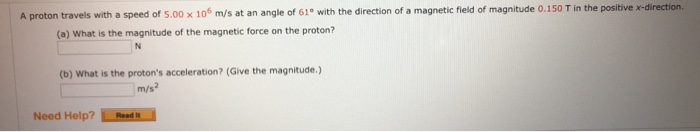A proton travels with a speed of 5.00 x 106 m/s at an angle of 61° with the direction of a magnetic field of magnitude 0.150 T in the positive x-direction. (a) What is the magnitude of the magnetic force on the proton? (b) What is the proton's acceleration? (Give the magnitude.) Need Help? Read

• ### a proton travels with a The City University of New York General Physics 2, Quiz 4...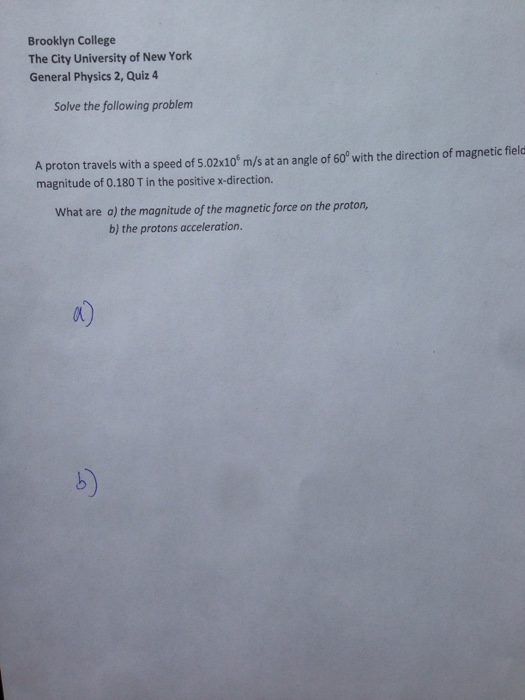a proton travels with a The City University of New York General Physics 2, Quiz 4 A proton travels with a speed of 5.02 times 10^6 m/s at an angle of 60 degree with the direction of magnetic field magnitude of 0.180 T in the positive x-direction. What are a) the magnitude of the magnetic force on the proton, the protons acceleration.

• ### 007 (part 1 of 2) 10.0 points A proton travels with a speed of 3.08 x...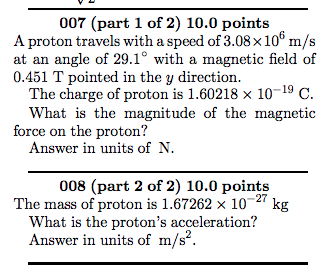007 (part 1 of 2) 10.0 points A proton travels with a speed of 3.08 x 10 m/s at an angle of 29.1° with a magnetic field of 0.451 T pointed in the y direction. The charge of proton is 1.60218 x 10-19 C. What is the magnitude of the magnetic force on the proton? Answer in units of N. 008 (part 2 of 2) 10.0 points The mass of proton is 1.67262 x 10-27 kg What is the proton's...

• ### 009 (part 1 of 3) 10.0 points A proton travels with a speed of 4.8×106 m/s...

009 (part 1 of 3) 10.0 points A proton travels with a speed of 4.8×106 m/s at an angle of 96◦ west of north. A magnetic field of 4.6 T points to the north. Find the magnitude of the magnetic force on the proton. (The magnetic force experienced by the proton in the magnetic field is proportional to the component of the proton’s velocity that is perpendicular to the magnetic field.) Answer in units of N. 010 (part 2 of...

• ### both questions this assignment, you submit answers by question parts. The number of submissions remaining for...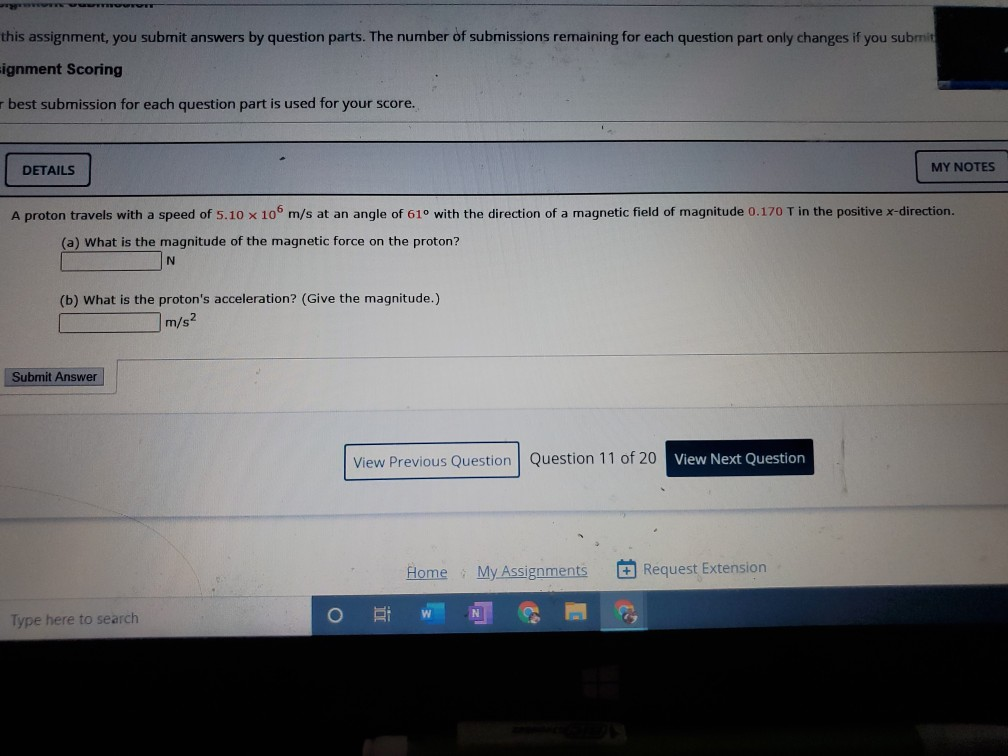both questions this assignment, you submit answers by question parts. The number of submissions remaining for each question part only changes if you submit Eignment Scoring best submission for each question part is used for your score. DETAILS MY NOTES A proton travels with a speed of 5.10 x 10 m/s at an angle of 61° with the direction of a magnetic field of magnitude 0.170 T in the positive x-direction. (a) What is the magnitude of the magnetic force...

• ### An alpha particle has a positive charge that is double the charge of a proton, and...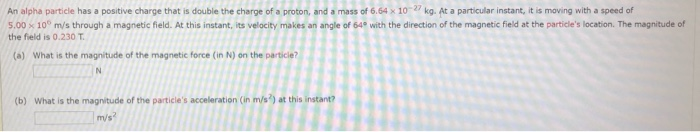An alpha particle has a positive charge that is double the charge of a proton, and a mass of 6.64 x 10- kg. At a particular instant, it is moving with a speed of 5.00 x 100 m/s through a magnetic field. At this instant, its velocity makes an angle of 64 with the direction of the magnetic field at the particle's location. The magnitude of the field is 0.230 T (a) What is the magnitude of the magnetic force...

• ### A proton moving at 7.00 x 106 m/s through a magnetic field of magnitude 2.6 T...

A proton moving at 7.00 x 106 m/s through a magnetic field of magnitude 2.6 T experiences magnetic force of magnitude 6.9x10-13 N. What is the angle between the proton's velocity and the field? 6.2° 76.3° 13.7° 44.9°

• ### A charged particle moving through a magnetic field at right angles to the field with a...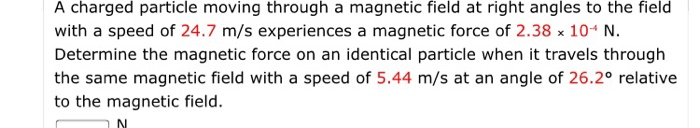A charged particle moving through a magnetic field at right angles to the field with a speed of 24.7 m/s experiences a magnetic force of 2.38 x 10* N. Determine the magnetic force on an identical particle when it travels through the same magnetic field with a speed of 5.44 m/s at an angle of 26.2° relative to the magnetic field. N What is the maximum force on a rod with a 0.100 C charge that you pass between the...